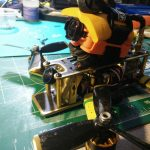# Goldy Specs (Jan 14th, 2016)

## Build

• Frame: Tweaker 180
• PDB: CC3D PDB (5v, 12v BECs)
• Flight Controller: SPRacingF3 with Betaflight 2.1.6
• Motors: DYS BE1806 2300KV
• ESCs: Lumenier 12 amp ESC w/ SimonK AutoShot  (Damped Light and Oneshot enabled)

## FPV

• Video TX: Eachine ET600 5.8G 32CH 600mW
• FPV Camera: Runcam SKYPLUS
• OSD: MinimOSD with MultiWii firmware

## Misc

• Battery: 4S LiPo MultiStar Race Spec 4S 1400mAh 40-80C or Lumenier 1000mAh 60C, 1300mAh 60C, 1500mAh 45CV
• Propellers: Usually 4045BN DAL or tri-blade
• HD Camera: Runcam2

## PIDs

LuxFloat PID Controller

```set pid_controller = LUX
set p_pitch = 40
set i_pitch = 30
set d_pitch = 18
set p_roll = 40
set i_roll = 30
set d_roll = 18
set p_yaw = 100
set i_yaw = 50
set d_yaw = 10
set p_pitchf = 1.700
set i_pitchf = 0.500
set d_pitchf = 0.015
set p_rollf = 1.500
set i_rollf = 0.500
set d_rollf = 0.013
set p_yawf = 5.000
set i_yawf = 0.300
set d_yawf = 0.005
set level_horizon = 6.000
set level_angle = 6.000
set sensitivity_horizon = 75
set p_alt = 50
set i_alt = 0
set d_alt = 0
set p_level = 50
set i_level = 50
set d_level = 100
set p_vel = 120
set i_vel = 45
set d_vel = 1
set gyro_soft_lpf = ON
set dterm_cut_hz = 40
set yaw_pterm_cut_hz = 50

# dump rates

# rateprofile
rateprofile 0

set rc_rate = 90
set rc_expo = 80
set rc_yaw_expo = 20
set thr_mid = 50
set thr_expo = 0
set roll_rate = 60
set pitch_rate = 60
set yaw_rate = 45
set tpa_rate = 33
set tpa_breakpoint = 1500```

## Media